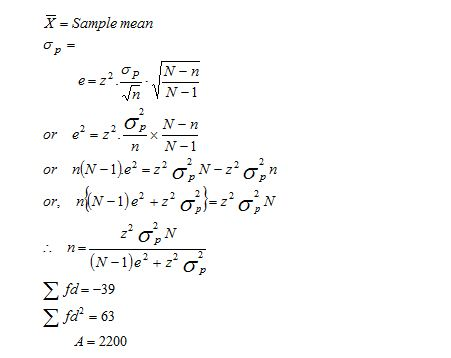# Lecture on VariableThe word variable is often used in the study of statistics, so it is important to understand its meaning. A variable is a characteristic that may assume more than one set of values to which a numerical measure can be assigned.

Height, age, amount of income, province or country of birth, grades obtained at school and type of housing are all examples of variables. Variables may be classified into various categories, some of which are outlined in this section.

What is meant by variable?

A variable is any trait that can change values from case to case.

Example: Income, age, gender

What is meant by data?

Data refer to any information express as numbers.

Example: 500, 70

What is meant by Descriptive Statistics ?

Descriptive statistics refers to a branch of statistics concerned with summarizing the distribution of a single variable or measuring the relationship between two or more variables.

What is meant by Inferential Statistics?

Inferential statistics refers to the branch of statistics concerned with making generalizations foe samples to populations.

What is meant by Statistics ?

Statistics is a set of mathematical techniques for organizing and analyzing data.

What is meant by Ratio Scale?

Ratio scales have an absolute or true zero of measurement. It represents the actual amounts of variables. Measure of physical dimensions such as weight, height, distance etc. are example / income , capital, labor etc. are also examples of ratio scale.Samples from Co.A

 Length of life (in hours) Midpoint f d fd fd2 f d fd fd2 1,700– 1,900 1800 10 –2 –20 40 3 –2 – 6 12 1,900–2,100 2000 16 –1 –16 16 40 –1 – 40 40 2,100–2,300 2200 20 0 0 0 12 0 0 0 2,300–2,500 2400 8 1 +8 8 3 1 +3 3 2,500–2,700 2600 6 2 +12 24 2 2 +4 8 N=60 d=0 Efd= –16 Efd2=88 N=60 d=0 Efd= –39 Efd2= 63

Here, A = 2200

i = 200